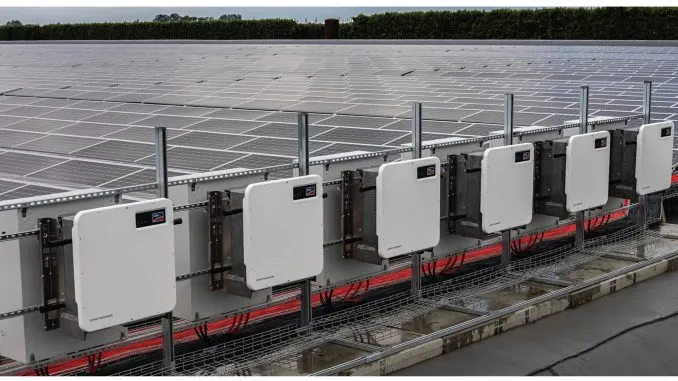Solair World

# How Much Does a Solar Inverter Cost?Solar inverters for a medium-sized setup typically range from \$1,000 to \$1,500. However, the cost can increase significantly with larger installations.

These costs can change based on market conditions, installation size, and specific inverter technology.

Cost Per Watt: The average cost of a solar inverter was about \$0.28 per watt. The price varied from as low as \$0.10 to as high as \$0.50 per watt.

Percentage of Total Installation Cost: Generally, the inverter makes up about 6% of the total cost of a solar installation. With an average installation cost at \$3.63 per watt, the inverter cost at \$0.28 per watt aligns with this percentage.

If the cost of your solar inverter represents more than 8% to 11% of the total installation cost, it’s advisable to discuss this with your installer.

Example Calculation for 6 kW Installation:

At the average rate of \$0.28 per watt, an inverter for a 6 kW system would cost around \$1,100.

If the inverter is priced at the higher end (\$0.50 per watt), the cost for the same system would be about \$1,650.

## Solar Inverter Cost by Types

Each type of inverter has its advantages and is suited for different solar system configurations.

The choice depends on various factors such as the size of the installation, specific energy needs, and environmental conditions.

When selecting an inverter, it’s important to consider not just the upfront cost but also the long-term benefits and efficiency of the system.

1. String Inverters:
• Cost Range: \$1,000 to over \$2,000.
• Price Factors: Determined by the inverter’s size and brand.
• Application: Commonly used in many solar installations.
2. Microinverters:
• Individual Cost: Around \$300 each.
• System Cost: Generally, a solar system with microinverters costs about 20% more than one with string inverters.
• Price Factors: Dependent on the number of solar panels and their output.
• Application: Ideal for systems where panels are partially shaded or face different directions.
3. Optimizers:
• Cost: Typically less expensive than microinverters. Adding optimizers to specific panels can cost a few hundred dollars.
• Application: Used for enhancing the efficiency of individual solar panels, particularly in partially shaded areas.
4. Hybrid Inverters:
5. Battery Inverters:
• Additional Cost: If a separate battery inverter is required, it can add \$2,000 to \$3,000 to the overall installation cost.
• Application: Necessary for solar systems with battery storage, especially if the main inverter doesn’t include battery integration.